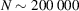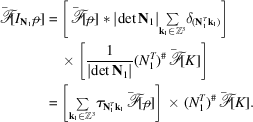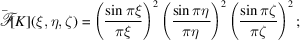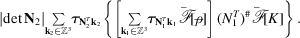International
Tables for
Crystallography
Volume B
Reciprocal space
Edited by U. Shmueli

International Tables for Crystallography (2010). Vol. B, ch. 1.3, pp. 92-93   | 1 | 2 |

## Section 1.3.4.4.3.4. Molecular averaging by noncrystallographic symmetries

G. Bricognea

aGlobal Phasing Ltd, Sheraton House, Suites 14–16, Castle Park, Cambridge CB3 0AX, England, and LURE, Bâtiment 209D, Université Paris-Sud, 91405 Orsay, France

#### 1.3.4.4.3.4. Molecular averaging by noncrystallographic symmetries

| top | pdf |

Macromolecules and macromolecular assemblies frequently crystallize with several identical subunits in the asymmetric metric unit, or in several crystal forms containing the same molecule in different arrangements. Rossmann & Blow (1963)recognized that intensity data collected from such structures are redundant (Sayre, 1952b) and that their redundancy could be a source of phase information.

The phase constraints implied by the consistency of geometrically redundant intensities were first derived by Rossmann & Blow (1963), and were generalized by Main & Rossmann (1966). Crowther (1967, 1969) reformulated them as linear eigenvalue equations between structure factors, for which he proposed an iterative matrix solution method. Although useful in practice (Jack, 1973), this reciprocal-space approach required computations of sizefor N reflections, so that N could not exceed a few thousands.

The theory was then reformulated in real space (Bricogne, 1974), showing that the most costly step in Crowther's procedure could be carried out much more economically by averaging the electron densities of all crystallographically independent sub­units, then rebuilding the crystal(s) from this averaged subunit, flattening the density in the solvent region(s) by resetting it to its average value. This operation is a projection [by virtue of Section 1.3.4.2.2.2(d)]. The overall complexity was thus reduced fromto N log N. The design and implementation of a general-purpose program package for averaging, reconstructing and solvent-flattening electron-density maps (Bricogne, 1976) led rapidly to the first high-resolution determinations of virus structures (Bloomer et al., 1978; Harrison et al., 1978), with.

The considerable gain in speed is a consequence of the fact that the masking operations used to retrieve the various copies of the common subunit are carried out by simple pointwise multiplication by an indicator functionin real space, whereas they involve a convolution within reciprocal space.

The averaging by noncrystallographic symmetries of an electron-density map calculated by FFT – hence sampled on a grid which is an integral subdivision of the period lattice – necessarily entails the interpolation of densities at nonintegral points of that grid. The effect of interpolation on the structure factors recalculated from an averaged map was examined by Bricogne (1976). This study showed that, if linear interpolation is used, the initial map should be calculated on a fine grid, of size Δ/5 or Δ/6 at resolution Δ (instead of the previously used value of Δ/3). The analysis about to be given applies to all interpolation schemes which consist in a convolution of the sampled density with a fixed interpolation kernel function K.

Letbe a-periodic function. Let K be the interpolation kernel in normalized' form, i.e. such thatand scaled so as to interpolate between sample values given on a unit grid; in the case of linear interpolation, K is the trilinear wedge'whereLetbe sampled on a grid, and letdenote the function interpolated from this sampled version of. Then:where, so thatThe transform ofthus consists of

 (i) a main band' corresponding to, which consists of the true transformattenuated by multiplication by the central region of; in the case of linear interpolation, for example,(ii) a series of ghost bands' corresponding to, which consist of translates ofmultiplied by the tail regions of.

Thusis not band-limited even ifis. Supposing, however, thatis band-limited and that gridsatisfies the Shannon sampling criterion, we see that there will be no overlap between the different bands:may therefore be recovered from the main band by compensating its attenuation, which is approximately a temperature-factor correction.

For numerical work, however,must be resampled onto another grid, which causes its transform to become periodized intoThis now causes the main bandto become contaminated by the ghost bandsof the translatesof.

Aliasing errors may be minimized by increasing the sampling rate in gridwell beyond the Shannon minimum, which rapidly reduces the r.m.s. content of the ghost bands.

The sampling rate in gridneeds only exceed the Shannon minimum to the extent required to accommodate the increase in bandwidth due to convolution with, which is the reciprocal-space counterpart of envelope truncation (or solvent flattening) in real space.

### References

Bloomer, A. C., Champness, J. N., Bricogne, G., Staden, R. & Klug, A. (1978). Protein disk of tobacco mosaic virus at 2.8 Ångström resolution showing the interactions within and between subunits. Nature (London), 276, 362–368.
Bricogne, G. (1974). Geometric sources of redundancy in intensity data and their use for phase determination. Acta Cryst. A30, 395–405.
Bricogne, G. (1976). Methods and programs for direct-space exploitation of geometric redundancies. Acta Cryst. A32, 832–847.
Crowther, R. A. (1967). A linear analysis of the non-crystallographic symmetry problem. Acta Cryst. 22, 758–764.
Crowther, R. A. (1969). The use of non-crystallographic symmetry for phase determination. Acta Cryst. B25, 2571–2580.
Harrison, S. C., Olson, A. J., Schutt, C. E., Winkler, F. K. & Bricogne, G. (1978). Tomato bushy stunt virus at 2.9 Ångström resolution. Nature (London), 276, 368–373.
Jack, A. (1973). Direct determination of X-ray phases for tobacco mosaic virus protein using non-crystallographic symmetry. Acta Cryst. A29, 545–554.
Main, P. & Rossmann, M. G. (1966). Relationships among structure factors due to identical molecules in different crystallographic environments. Acta Cryst. 21, 67–72.
Rossmann, M. G. & Blow, D. M. (1963). Determination of phases by the conditions of non-crystallographic symmetry. Acta Cryst. 16, 39–45.
Sayre, D. (1952b). Some implications of a theorem due to Shannon. Acta Cryst. 5, 843.